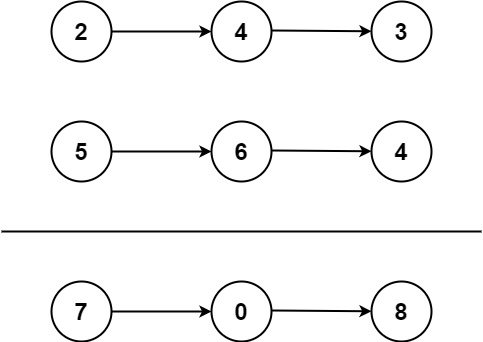# 2. Add Two Numbers / Medium

You are given two non-empty linked lists representing two non-negative integers. The digits are stored in reverse order, and each of their nodes contains a single digit. Add the two numbers and return the sum as a linked list.

You may assume the two numbers do not contain any leading zero, except the number 0 itself.

## Example 1:Input: l1 = [2,4,3], l2 = [5,6,4]
Output: [7,0,8]
Explanation: 342 + 465 = 807.

## Example 2:

Input: l1 = , l2 = 
Output: 

## Example 3:

Input: l1 = [9,9,9,9,9,9,9], l2 = [9,9,9,9]
Output: [8,9,9,9,0,0,0,1]

## Constraints:

• The number of nodes in each linked list is in the range `[1, 100]`.
• `0 <= Node.val <= 9`
• It is guaranteed that the list represents a number that does not have leading zeros.

# Solution

## 效能

### Complexity

• Time Complexity: O(M+N), where M and N is the length of list1 and list2 respectively.
• Space Complexity: O(1)

### LeetCode Result

• Runtime: 40 ms
• Memory Usage: 70.9 MB